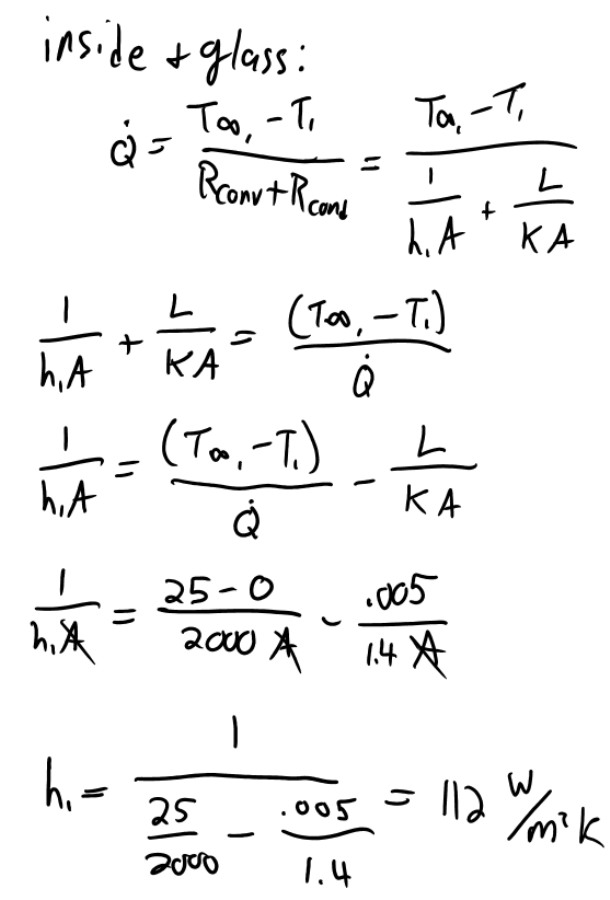heat and mass transfer problems engineering equations heat and mass transfer school homework engineering heat and mass transfer formulas heat and mass transfer solutions to heat and mass transfer problems full solution engineering problem solution heat and mass transfer math problems engineering equations heat and mass transfer school homework engineering solutions to heat and mass transfer formulas heat problems mass problem solutions to transfer problems full solution heat and mass transfer
heat and mass transfer problems engineering equations heat and mass transfer school homework engineering heat and mass transfer formulas heat and mass transfer solutions to heat and mass transfer problems full solution engineering problem solution heat and mass transfer math problems engineering equations heat and mass transfer school homework engineering solutions to heat and mass transfer formulas heat problems mass problem solutions to transfer problems full solution heat and mass transfer
Highalphabet Home Page heat and mass transfer problem solutions Heat and Mass Transfer Page
To defrost ice accumulated on the outer surface of an automobile windshield, warm air is blown over the inner surface of the windshield. Consider an automobile windshield with thickness of 5 mm and thermal conductivity of 1.4 W/mK. The outside ambient temperature is -10 C and the convection heat transfer coefficient is 200 W/m^2 K, while the ambient temperature inside the automobile is 25 C. Determine the value of the convection heat transfer coefficient for the warm air blowing over the inner surface of the windshield necessary to cause the accumulated ice to begin melting.To defrost ice accumulated on the outer surface of an automobile windshield, warm air is blown over the inner surface of the windshield. Consider an automobile windshield with thickness of 5 mm and thermal conductivity of 1.4 W/mK. The outside ambient temperature is -10 C and the convection heat transfer coefficient is 200 W/m^2 K, while the ambient temperature inside the automobile is 25 C. Determine the value of the convection heat transfer coefficient for the warm air blowing over the inner surface of the windshield necessary to cause the accumulated ice to begin melting.